Resources Section

Web Application for Symmetrical Componets: http://personal.strath.ac.uk/steven.m.blair/seq/

Do you have a question? Click on the "Questions & Answers forum" and ask away!

This video was brought to you by GeneralPAC.com, making power systems Intuitive, Open and Free for Everyone, Everywhere. Consider subscribing and supporting through patreon.com/GeneralPAC. This is a mechanism for you to support us financially so we can continue making high quality power system video tutorials. Our corporate sponsor for this topic is AllumiaX.com from Seattle, Washington. Contact them for industrial and commercial power system studies.

Principles of Symmetrical Components part three E. In part three E, we're going to use some of the concepts of symmetrical components that we learn in the previous tutorial and we're going to take positive sequence component, negative sequence component and zero sequence components and add them up to see if we get our original phasor. Before we get into it, we should really understand exactly what we're dealing with. Okay. What we have here is an unbalanced set of three phases. Phase A current, right, we'll call this current. Phase A current is the red phasor. Phase B current is the yellow phasor and phase C current is the blue phasor. So, if all three phasors were rotating in the counter clockwise direction, it would make an A, B, C phase sequence. Okay.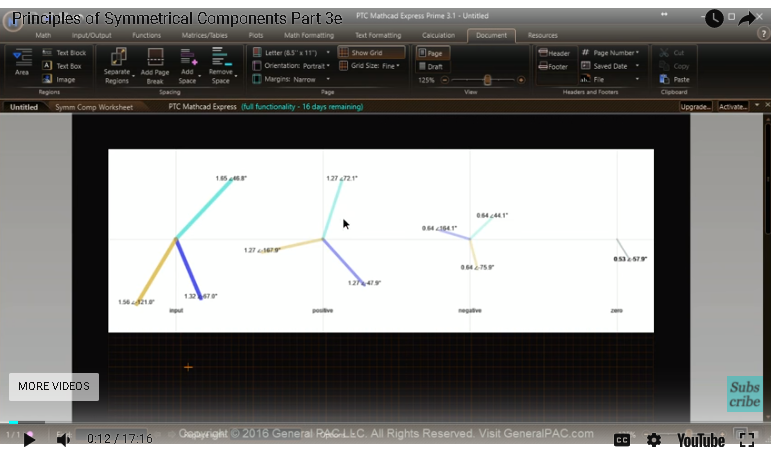But, as you can see, this is an unbalanced set, because the magnitude of all three phases do not equal one another and the angle displacement between the phasors are not 120 degrees. So, this is an unbalanced set and because this is an unbalanced set, if we take the symmetrical components of this unbalanced set, we're going to get positive sequence components, negative sequence components and zero sequence components. But, in this tutorial, we're going to take these positive, negative and zero sequence components as they are given and we're going to use the concepts that we've learned to see if we can mathematically represent these symmetrical components back into the original system phasor. Okay.

So, that's our goal. We're going to take positive, negative, zero sequence component and see if we can represent the original phasor through mathematics. Okay, so, let's get started.

So, first, let's define our symmetrical component phasors, and we're going to say that the positive sequence component I positive sequence component for phasor A, that is equal to 1.27, right, which is this guy right here. 1.27 at the angle of 72.1 degrees. An angle of 72.1 degrees. Okay. So, now we've defined IA positive sequence component for phase A. Now, we have to do the same thing for phase B. So, we know that the positive sequence component for phase B, which is this guy right here, that is equal to 1.27 at the angle of negative 47.9 degrees and then lastly the positive sequence component for phase C current is equal to 1.27 at the angle of negative 167.9 degrees.

So, now we've defined the positive sequence component. Now, let's define the negative sequence component. So, we're gonna scroll down a little bit. Let's zoom out. Okay. So, now, we're dealing with the negative sequence component. So, the negative sequence component of phase A, which is the red. So, that is equal to 0.64 at 44.1 degrees. Let's go back in here. So, we're gonna say 0.64 at 44.1 degrees and then for simplicity, let's just copy that below, so we're not retyping everything. So, for phase B, now phase B was this one right here, that is equal to same magnitude, but at negative 75.1 degrees. Negative 75.1 degrees and this is for phase B. Okay.

Then for phase C, which is the yellow, same magnitude, but it's at 164.1 degrees, so phase C. 64.1 degrees. Awesome. Now, lastly, let's do the same thing for zero sequence component. So, for zero sequence component, zero A, we know that phase A, phase B and phase C all have the same magnitude and they all have the same angle and the reason why we can't see the other two phases here and the reason why we only have this one line is because all three phases overlap each other. So, for the zero sequence component, all three phases are .53 at negative 57.9 degrees. So, that's what we're going to write, so .53 at negative 57.9 degrees. Okay, so before we go on to the next page, let's audit some of our values and make sure we have everything correct because if it's not correct, then it's gonna cause more problems later on.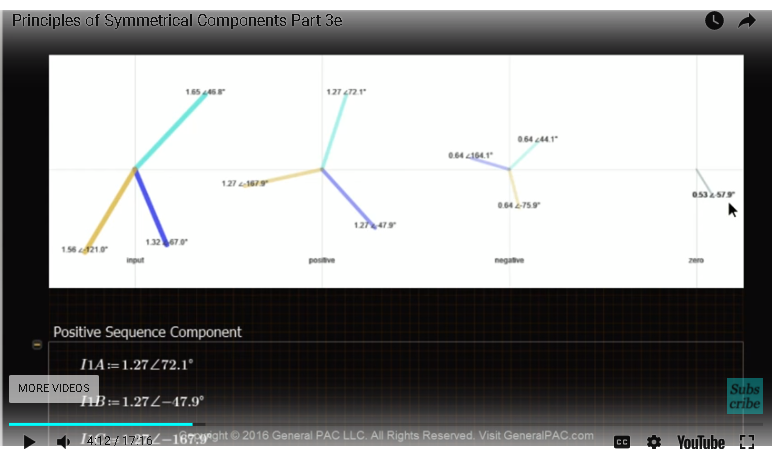So, positive sequence phase A component is 1.27 right here at 72.1 degrees, which is right. For phase B, is 1.27 at negative 47.9 degrees, which is okay and then for phase C is 1.27 at negative 167.9 degrees, which is cool. So, I'm going to zoom out a little bit. For negative sequence component phase A, which is the red, is .64 at 44.1 degrees, which is what we have here. That's good. For phase B, phase B was a yellow. It's 0.64 at 164.1 degrees and oh no, we have a mistake here. So, and then, so, this right here is actually 164.1 degrees. So, phase B, which is the yellow is 164.1 degrees and it's not negative. It's positive and then for phase C, which is the blue. It's .64 at negative 75.9 degrees. So, phase C is negative 75.9 degrees.

Okay, and then our zero sequence components, .53 at negative 57.9 degrees. .53 at negative 57.9 degrees. Okay, we are looking good now. As you can see, I had made a rookie mistake of swapping phase B and C for negative sequence component. So, now, let's go on to the second page and start doing our mathematics and see if we can get our original phasor. So, let's copy this guy into our second page, so we don't have to scroll back and forth. Increase the size of this. Okay, so, remember that the red phasor was phase A? In the previous tutorial we said that the system phase A phasor is equal to the phase A positive sequence component plus phase A negative sequence component plus phase A zero sequence component right? Well, let's see if that works out. So, the system phasor, right, that is equal to positive sequence component for phase A. The negative sequence component for phase A and the zero sequence component for phase A.

Let's see if it works and we have to change the format and there you have it. 1.653 at 46.8 degrees. Which is great because we know that if we add a positive sequence component, negative sequence component, zero sequence component, we get the original phasor. Awesome. Okay, so this is great. Now, let's do IB. So, IB is equal to the positive sequence component for phase B plus the negative sequence component for phase B plus the zero sequence component for phase B and let's see if it equals our original phasor and there you have it. Remember we said that phase B was a yellow phasor? 1.32 at negative 67 degrees and that's what we have here.

Okay. This is looking great. Now, the original C, system phasor is equal to positive sequence component phase C plus the negative sequence component phase C plus the zero sequence component of phase C and that is equal to 1.56 at negative 121 degrees. 1.56 at negative 121 degrees and that equals our original system phasor. So, we just used some of the mathematics to equate our original phasor. Right? Now, this is just one way of equating the original phasor with the symmetrical component breakdowns. Let's investigate another way. So, let's define our A operator.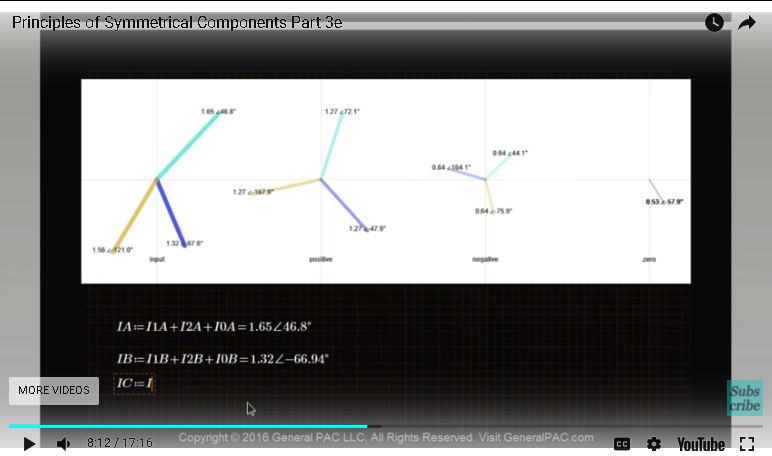So, let's say that our A operator is equal to one at the angle of 120 degrees. Okay, which means that A superscript or A squared, that is equal to one at negative 120 degrees, so don't get confused. This is exactly how we defined it in the past. So, we're going to calculate the original phasor A, but we're using the second method and that is equal to, well we're gonna put zero sequence component first, right. So, zero sequence component phase A plus the positive sequence component phase A plus the negative sequence component phase A and that is equal to our original phasor. Right, which make sense because it equals that. Now, we're saying IB original phasor using the second method is equal to I zero B, plus now, we're gonna use I 1A. So, here we have to use the positive sequence component for phase B. Right, which is this guy right here. The yellow.

So, positive sequence component for phase B is yellow. We have to use that, but instead, we're gonna take advantage of the A operators and take the phase A positive sequence and just rotate that 120 degrees in the counter clockwise direction or rotate that 240 degrees in the clockwise direction. So, we're gonna take IA and we're gonna multiply that by A superscript two and then for the negative sequence component, which is this guy here, we have to use I phase B, right. We have to use phase B, which is the yellow. Right. But, instead of using phase B explicitly, let's use it implicitly by taking phase A and rotating that 120 degrees in the clockwise direction. So, we're gonna take I negative sequence component of A and then we're just going to rotate that 120 degrees in a clockwise direction and let's see if we get our original phasor.

And there you have it. So, 1.322 at negative 66.94 degrees and remember that this was our original phasor. Look at here. It's 1.32 at negative 67 degrees. So, we do in fact, get our original phasors by simply using these A operators instead. So, now let's do the same thing with the IC original phasor. So, now we're going to have, so IC original phasor. Second method is equal to zero sequence component of IC plus, by the way, before we continue this, notice that if I change the zero sequence component here to just A, we'll get the same answer because the zero sequence components are all equal to each other and they have the same phase angle. So, similarly, we could represent this as phase A zero sequence component. Okay. Now, the positive sequence component, now we have to use phase C. Right.

So, phase C was the blue, so now we can take phase A and then we can rotate that 120 degrees to make it equal with phase C. So, we'll take IA positive component for phase A and then we'll rotate that by the A operator and then similarly, for negative sequence component, here is the negative sequence component. Here is phase C. Remember blue is phase C. We're going to take the negative sequence component and then rotate that 240 degrees in the counter clockwise direction to represent phase C or we can take phase A and we can rotate it negative 120 degrees in the clockwise direction and we'll get the same phasor. Okay.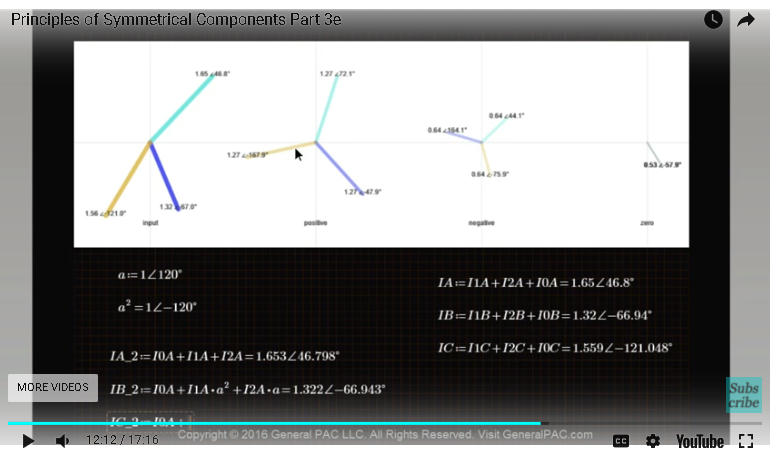So, essentially, what we're saying is we have to use A squared operator or A superscript two operator and let's see if we get the same answer. So, phase C, which is the blue. 1.56 at negative 121 degrees. 1.56 at negative 121 degrees and that all makes sense, right. So, this, we got the same answer using the second method, which is great.

Okay. Now, let's use this third method. Now, the third method is a lot easier and what we're going to so is going to use the matrix form. Okay. So, here's a matrix form, so we're going to add in a one by three matrix here right and we're gonna say that that is equal to another matrix that's three by three and that is multiplied by another matrix that's one by three. So, we're gonna put in IA. Now this is the third method, right. IB and IC and then in here, we plug in our matrices that we've developed in the past. So, we know that this is A superscript two. This is A. This is A and this is A superscript two.

All right, and then we plug in our reference symmetrical component for phase A, which is the positive sequence component for phase A. The negative sequence component for phase A and the zero sequence component for phase A and let's see if that matches what our original answers were. So, we'll convert that. Here is the third method. So, the original phasor for phase A, 1.653 at 45, about 46 degrees right here, which makes sense. Phase B, 1.32. 1.32 at 53 degrees. All right. We have a problem. Okay. We have a problem. So, what is our problem? I think I know what our problem is. So, remember when we defined this third column here? It's very, very important to put the zero sequence component first. Okay. The zero sequence component goes in first and then it's the positive sequence component and the negative sequence component.

Okay, so zero sequence component first, then positive then negative, right. If we want to use the positive first, and the negative second and the zero third, then we have to rearrange this matrix here. So, this form right here is specifically for zero sequence component being first, then the positive sequence being second and the negative sequence being third. And once we've done that, look what happened. So, for phase A it's 1.653 at 46.8 degrees, which makes sense. For phase B, it's 1.32. 1.32 at negative 66.9 degrees or 67 degrees and then for phase C, it's 1.56 at negative 121 degrees, so phase C is 1.56 at negative 121 degrees. So, in this tutorial, though it was very long, we've went through multiple methods to mathematically calculate our original phasor using symmetrical component and this concludes the part three session.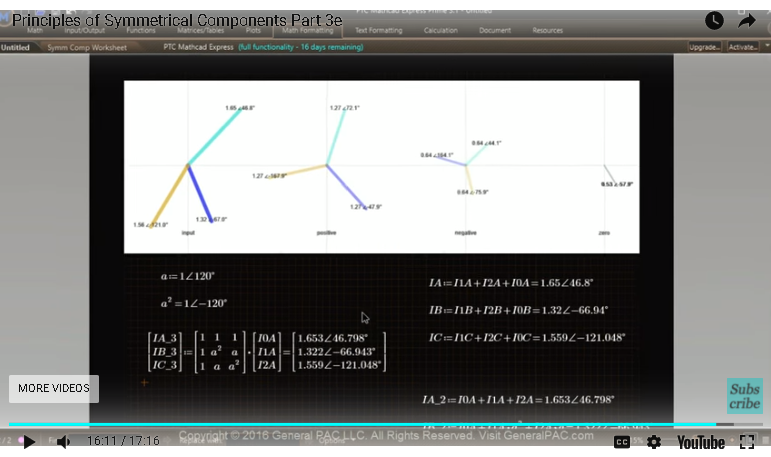In part four, we're going to work backwards to look at, okay, so if we had this original phasor and it was unbalanced, how can we calculate the positive and negative and zero sequence component from this unbalanced set? Right. In part three, we focused on, okay, we have positive, negative, zero sequence components. How do we calculate the systems symmetrical component in part four? We had the system symmetrical component, how do we calculate the positive, negative, zero sequence components like that. This video was brought to you by GeneralPack.com making power system protection, automation and controls intuitive. Please visit GeneralPack.com for more power systems, video tutorials similar to this one and subscribe to this channel.

Thank you.

Greetings from the GeneralPAC Team!

We make high-quality Power Systems Video Tutorials on complex topics that are free and open to everyone!  Thank you so much for supporting us through Patreon so can continue doing good and valuable work.

What is Patreon and why do we use it?

Patreon is a fantastic portal that allows our fans and community to make monthly contribution (like Netflix subscription) so we can continue creating high-quality power systems video tutorials. In return, you get access to incredible perks like voting on future topics, getting your questions answered, access to VIP Q/A webinars with the creators of GeneralPAC, and much more! We THANK YOU for supporting us

Why do we need your support?

An incredible amount of time and effort is needed to develop high-quality video tutorials. Each video (Part 1 for example) takes approximately 10 hours to complete which includes learning the concept ourselves, brainstorming creative ways to teach and explain the concepts, writing the script, audio recording, video recording, and editing. It's no wonder why Hundreds-of-Thousands of people have watched, liked, subscribed, and left positive comments on Youtube channel. Your support truly makes all the difference.